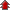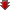Home Board Paper Solutions Text Book Solutions Articles NCERT Exemplar Solutions

# Solutions### Exercise 1

•  Q1 Calculate the mass percentage of benzene (C6H6) and carbon tetrachloride (CCl4) if 22 g of benzene is dissolved in 122 g of carbon tetrachloride. Q2 Calculate the mole fraction of benzene in solution containing 30% by mass in carbon tetrachloride. Q3 Calculate the molarity of each of the following solutions: (a)30 g of Co(NO3)2. 6H2O in 4.3 L of solution (b)30 mL of 0.5 M H2SO4 diluted to 500 mL. Q4 Calculate the mass of urea (NH2CONH2) required in making 2.5 kg of 0.25 molal aqueous solution. Q5 Calculate (a) molality (b) molarity and (c) mole fraction of KI if the density of 20% (mass/mass) aqueous KI is 1.202 g mL-1. Q6 H2S, a toxic gas with rotten egg like smell, is used for the qualitative analysis. If the solubility of H2S in water at STP is 0.195 m, calculate Henry's law constant.">H2S, a toxic gas with rotten egg like smell, is used for the qualitative analysis. If the solubility of H2S in water at STP is 0.195 m, calculate Henry's law constant. Q7 Henry's law constant for CO2 in water is 1.67 x 108Pa at 298 K. Calculate the quantity of CO2in 500 mL of soda water when packed under 2.5 atm CO2 pressure at 298 K.">Henry's law constant for CO2 in water is 1.67 x 108Pa at 298 K. Calculate the quantity of CO2in 500 mL of soda water when packed under 2.5 atm CO2 pressure at 298 K. Q8 The vapour pressure of pure liquids A and B are 450 and 700 mm Hg respectively, at 350 K. Find out the composition of the liquid mixture if total vapour pressure is 600 mm Hg. Also find the composition of the vapour phase.">The vapour pressure of pure liquids A and B are 450 and 700 mm Hg respectively, at 350 K. Find out the composition of the liquid mixture if total vapour pressure is 600 mm Hg. Also find the composition of the vapour phase. Q9 Vapour pressure of pure water at 298 K is 23.8 mm Hg. 50 g of urea (NH2CONH2) is dissolved in 850 g of water. Calculate the vapour pressure of water for this solution and its relative lowering.">Vapour pressure of pure water at 298 K is 23.8 mm Hg. 50 g of urea (NH2CONH2) is dissolved in 850 g of water. Calculate the vapour pressure of water for this solution and its relative lowering. Q10 Boiling point of water at 750 mm Hg is 99.63°C. How much sucrose is to be added to 500 g of water such that it boils at 100°C.Molal elevation constant for water is 0.52 K kg mol-1. Q11 Calculate the mass of ascorbic acid (Vitamin C, C6H8O6) to be dissolved in 75 g of acetic acid to lower its melting point by 1.5°C. Kf= 3.9 K kg mol-1.">Calculate the mass of ascorbic acid (Vitamin C, C6H8O6) to be dissolved in 75 g of acetic acid to lower its melting point by 1.5°C. Kf= 3.9 K kg mol-1. Q12 Calculate the osmotic pressure in pascals exerted by a solution prepared by dissolving 1.0 g of polymer of molar mass 185,000 in 450 mL of water at 37°C.">Calculate the osmotic pressure in pascals exerted by a solution prepared by dissolving 1.0 g of polymer of molar mass 185,000 in 450 mL of water at 37°C.## Recently Viewed Questions of Class 12th chemistry

• NCERT Chapter